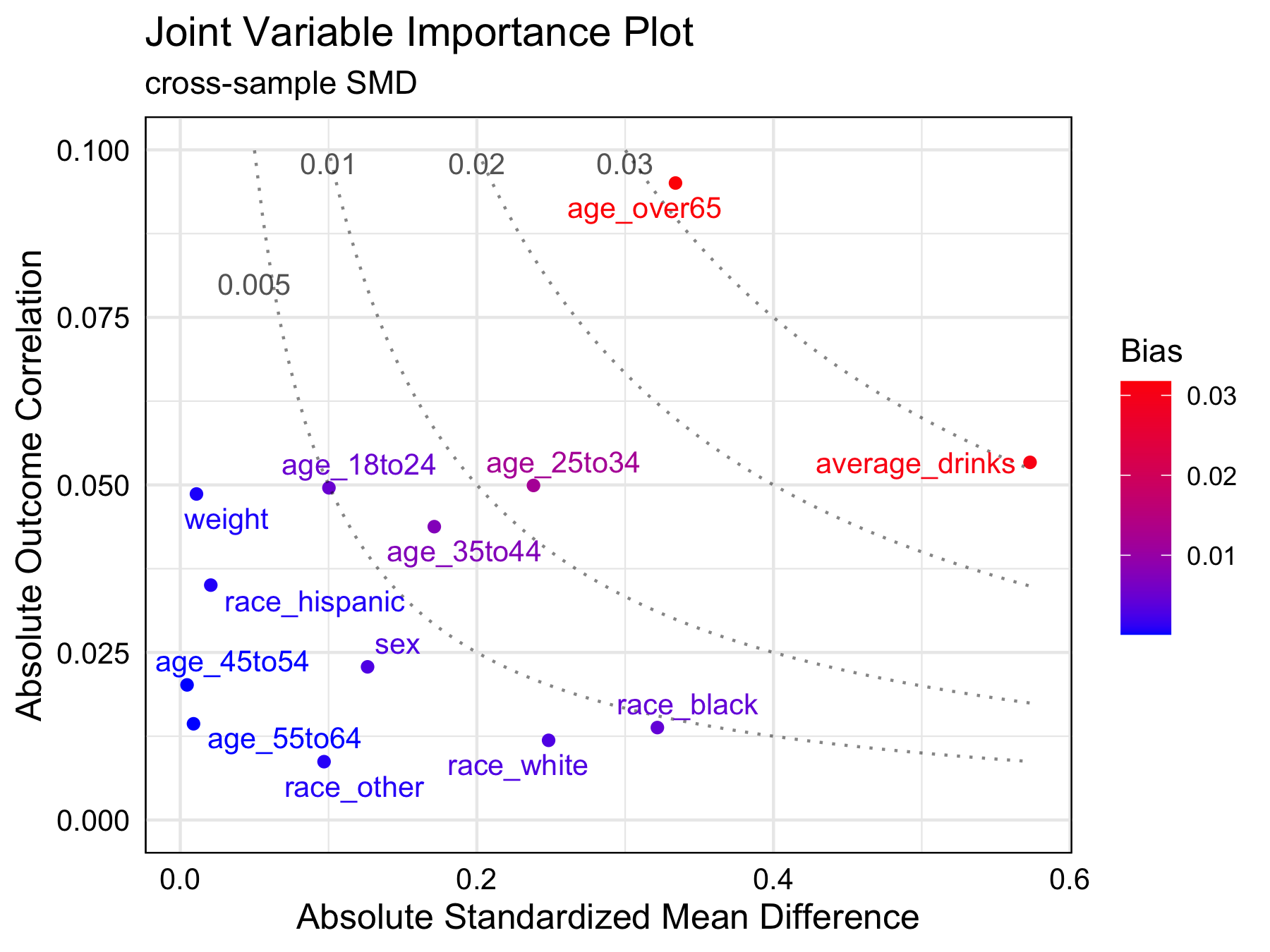# Joint variable importance plotJoint variable importance plot (jointVIP) visualizes each variable’s outcome importance via Pearson’s correlation and treatment importance via cross-sample standardized mean differences. Bias curves enable comparisons to support prioritization.

## Installation

You can install the `jointVIP` package on CRAN using:

``````# for version on CRAN
install.packages("jointVIP")

# for development version on github
devtools::install_github("ldliao/jointVIP")``````

## BRFSS Example

To demonstrate, we use the 2015 Behavioral Risk Factor Surveillance System (BRFSS) example to answer the causal question: Does smoking increase the risk of chronic obstructive pulmonary disease (COPD)? The data and background is inspired by Clay Ford’s work from University of Virginia Library. First, the data is cleaned to only have numeric variables, i.e., all factored variables are transformed via one-hot-encoding. Treatment variable `smoke` only contains 0 (control) and 1 (treatment).

With the cleaned data, you can specify details in the function `create_jointVIP()` like so:

``````library(jointVIP)
## basic example code

treatment = 'smoke'
outcome = 'COPD'
covariates = names(df)[!names(df) %in% c(treatment, outcome)]

## select the pilot sample from random portion
## pilot data here are considered as 'external controls'
## can be a separate dataset; should be chosen with caution
set.seed(1234895)
pilot_prop = 0.2
pilot_sample_num = sample(which(df %>% pull(treatment) == 0),
length(which(df %>% pull(treatment) == 0)) *
pilot_prop)

## set up pilot and analysis data
## we want to make sure these two data are non-overlapping
pilot_df = df[pilot_sample_num, ]
analysis_df = df[-pilot_sample_num, ]

## minimal example
brfss_jointVIP = create_jointVIP(treatment = treatment,
outcome = outcome,
covariates = covariates,
pilot_df = pilot_df,
analysis_df = analysis_df)``````

Generic functions can be used for the `jointVIP` object to extract information as a glance with `summary()` and `print()`.

``````summary(brfss_jointVIP)
#> Max absolute bias is 0.032
#> 3 variables are above the desired 0.01 absolute bias tolerance
#> 13 variables can be plotted
print(brfss_jointVIP)
#>                 bias
#> age_over65     0.032
#> average_drinks 0.031
#> age_25to34     0.012``````
``plot(brfss_jointVIP)``In this example, `age_over65` and `average_drinks` are two most important variables to adjust. At a bias tolerance of 0.01, 3 variables: `age_over65`, `average_drinks`, and `age_25to34` are above the tolerance threshold. Moreover, `age_over65` and `average_drinks` are of higher importance for adjustment than `age_25to34`. Although `race_black` and `age_over65` have similar absolute standardized mean differences (0.322 and 0.333, respectively), `age_over65` is more important to adjust for since its highly correlated with the outcome.